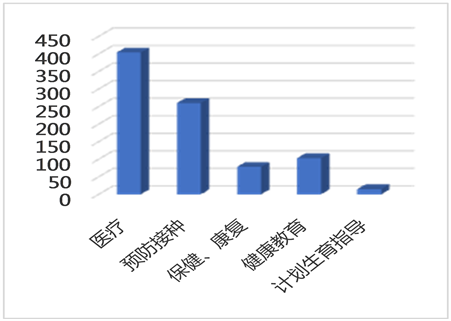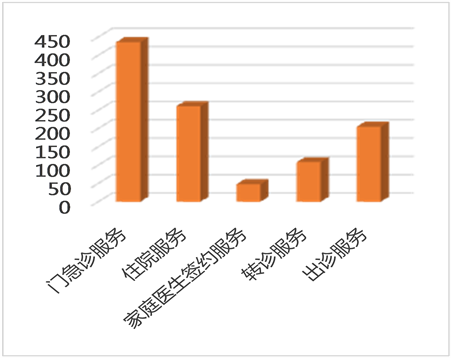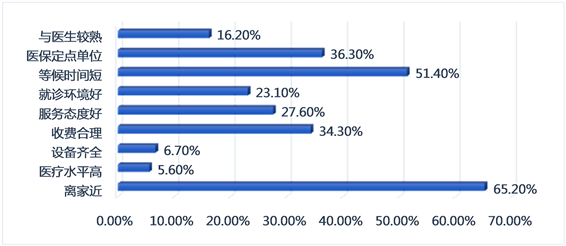# 社区公共卫生服务质量评价研究——以西安市为例Research on the Evaluation of Community Public Health Service Quality—Taking Xi’an as an Example

DOI: 10.12677/ASS.2020.97151, PDF, HTML, XML, 下载: 29  浏览: 89  国家社会科学基金支持

Abstract: Based on customer perception of service quality theory and SERVQUAL evaluation model, this article combined the actual situation to construct the original indicators for evaluating the quality of community public health services, selected the indicators through two rounds of expert consultation, and used AHP to weight each indicator. The study adopted stratified sampling method to conduct an empirical study on 468 patients from 6 community public health service centers in Beilin and Lianhu districts with high population density. The results showed that the community public health services of Xi’an have achieved certain results in the construction of tangible environment, responsiveness of diagnosis and treatment, and assurance of service attitude, but the scores in regard of the assurance during the diagnosis and treatment process and the empathy of service are low. So the government should further improve the medical technology level of community doctors, actively implement empathy services such as family contracting, and enhance the community’s public health service capabilities, thereby transforming the residents’ “inverted-triangle” medical order.

1. 引言

2. 资料与方法

2.1. 调查对象

2019年6~8月对西安市2个老城区各随机抽取3家社区公共卫生服务中心，以在这6家社区公共卫生服务中心就医的患者为调查对象，受调查者必须年满18周岁，能理解调查问卷的内容并愿意配合调查。

2.2. 调查方法

2.3. 统计学方法

3. 数据分析

3.1. 调查对象基本情况

1) 样本的性别、年龄受教育程度分析

2) 患者职业、医疗费用负担方式及年人均医疗支出

3.2. 社区公共卫生服务认知及利用分析Figure 1. Service project cognitionFigure 2. Service mode cognitionFigure 3. Analysis of reasons for community visits

3.3. 社区公共卫生服务模糊综合评价分析

1) 确定评价因素集

U1 = {医务人员着装举止、空间布局、设施配备、环境卫生}、U2 = {医务人员技术水平、诊疗服务过程安全、诊疗方案的精准、诊疗记录准确}、U3 = {诊疗方案安排及时、患者意见的处理、诊疗服务流程合理、排队等候时间合理、双向转诊及时}、U4 = {医务人员服务态度、对患者的尊重程度、如实告知诊疗结果、隐私保护、患者就医时感到放心}、U5 = {医护人员与患者主动沟通交流、医护人员会根据实际情况提供合理的诊疗方案、健康教育、家庭签约服务、特殊人群个性化服务}。

2) 建立评价集

3) 确定评价指标权重集Table 1. Judgment matrix and weight of Xi’an community public health service quality evaluation criteria layerTable 2. Judgment matrix and weight of tangible quality of scheme layerTable 3. Judgment matrix and weight of reliability quality of scheme layerTable 4. Judgment matrix and weight of responsiveness quality of scheme layerTable 5. Judgment matrix and weight of assurance quality of scheme layerTable 6. Judgment matrix and weight of empathy quality of scheme layerTable 7. Community public health service quality evaluation index system

4) 建立模糊综合评价矩阵

$R={\left({r}_{ij}\right)}_{m}{}_{×n}=\left[\begin{array}{cccc}{r}_{11}& {r}_{12}& \cdots & {r}_{1n}\\ {r}_{21}& {r}_{22}& \cdots & {r}_{2n}\\ ⋮& ⋮& \ddots & ⋮\\ {r}_{m1}& {r}_{m2}& \cdots & {r}_{mn}\end{array}\right]$

${R}_{1}=\left[\begin{array}{cc}\begin{array}{cc}\begin{array}{c}0.162\\ 0.105\end{array}& \begin{array}{c}0.402\\ 0.404\end{array}\\ 0.062& 0.286\end{array}& \begin{array}{ccc}0.423& 0.013& 0\\ 0.434& 0.036& 0.021\\ 0.502& 0.103& 0.047\end{array}\\ \begin{array}{cc}0.081& 0.382\end{array}& \begin{array}{ccc}0.470& 0.021& 0.045\end{array}\end{array}\right]$

${R}_{2}=\left[\begin{array}{cc}\begin{array}{cc}\begin{array}{c}0.026\\ 0.062\end{array}& \begin{array}{c}0.271\\ 0.362\end{array}\\ 0.062& 0.323\end{array}& \begin{array}{ccc}0.541& 0.092& 0.071\\ 0.482& 0.081& 0.013\\ 0.506& 0.092& 0.017\end{array}\\ \begin{array}{cc}0.049& 0.350\end{array}& \begin{array}{ccc}0.496& 0.096& 0.009\end{array}\end{array}\right]$

${R}_{3}=\left[\begin{array}{cc}\begin{array}{cc}\begin{array}{c}0.062\\ 0.075\end{array}& \begin{array}{c}0.410\\ 0.397\end{array}\\ 0.075& 0.432\end{array}& \begin{array}{ccc}0.462& 0.043& 0.024\\ 0.466& 0.043& 0.019\\ 0.427& 0.066& 0\end{array}\\ \begin{array}{cc}\begin{array}{c}0.085\\ 0.085\end{array}& \begin{array}{c}0.404\\ 0.389\end{array}\end{array}& \begin{array}{ccc}\begin{array}{c}0.432\\ 0.451\end{array}& \begin{array}{c}0.051\\ 0.049\end{array}& \begin{array}{c}0.028\\ 0.026\end{array}\end{array}\end{array}\right]$

${R}_{4}=\left[\begin{array}{cc}\begin{array}{cc}\begin{array}{c}0.098\\ 0.107\end{array}& \begin{array}{c}0.453\\ 0.444\end{array}\\ 0.062& 0.455\end{array}& \begin{array}{ccc}0.365& 0.068& 0.015\\ 0.368& 0.081& 0\\ 0.389& 0.058& 0.036\end{array}\\ \begin{array}{cc}\begin{array}{c}0.096\\ 0.068\end{array}& \begin{array}{c}0.370\\ 0.400\end{array}\end{array}& \begin{array}{ccc}\begin{array}{c}0.449\\ 0.436\end{array}& \begin{array}{c}0.085\\ 0.090\end{array}& \begin{array}{c}0\\ 0.006\end{array}\end{array}\end{array}\right]$

${R}_{5}=\left[\begin{array}{cc}\begin{array}{cc}\begin{array}{c}0.075\\ 0.064\end{array}& \begin{array}{c}0.410\\ 0.387\end{array}\\ 0.060& 0.327\end{array}& \begin{array}{ccc}0.440& 0.043& 0.032\\ 0.455& 0.068& 0.026\\ 0.474& 0.109& 0.030\end{array}\\ \begin{array}{cc}\begin{array}{c}0.062\\ 0.092\end{array}& \begin{array}{c}0.291\\ 0.295\end{array}\end{array}& \begin{array}{ccc}\begin{array}{c}0.564\\ 0.509\end{array}& \begin{array}{c}0.045\\ 0.083\end{array}& \begin{array}{c}0.038\\ 0.021\end{array}\end{array}\end{array}\right]$

5) 综合评价

$\begin{array}{c}{B}_{1}={W}_{1}\ast {R}_{1}\\ =\left(0.0535,0.1424,0.5868,0.2172\right)\left[\begin{array}{cc}\begin{array}{cc}\begin{array}{c}0.066\\ 0.049\end{array}& \begin{array}{c}0.502\\ 0.491\end{array}\\ 0.062& 0.310\end{array}& \begin{array}{ccc}0.426& 0.006& 0\\ 0.436& 0.011& 0.013\\ 0.515& 0.081& 0.032\end{array}\\ \begin{array}{cc}0.053& 0.485\end{array}& \begin{array}{ccc}0.444& 0.015& 0.003\end{array}\end{array}\right]\\ =\left(0.0584,0.3840,0.4835,0.0527,0.0213\right)\end{array}$

$\begin{array}{c}{B}_{2}={W}_{2}\ast {R}_{2}\\ =\left(0.5577,0.2594,0.1124,0.0705\right)\left[\begin{array}{cc}\begin{array}{cc}\begin{array}{c}0.026\\ 0.062\end{array}& \begin{array}{c}0.271\\ 0.362\end{array}\\ 0.062& 0.323\end{array}& \begin{array}{ccc}0.541& 0.092& 0.071\\ 0.482& 0.081& 0.013\\ 0.506& 0.092& 0.017\end{array}\\ \begin{array}{cc}0.049& 0.350\end{array}& \begin{array}{ccc}0.496& 0.096& 0.009\end{array}\end{array}\right]\\ =\left(0.0408,0.3063,0.5184,0.0894,0.0452\right)\end{array}$

$\begin{array}{c}{B}_{3}={W}_{3}\ast {R}_{3}\\ =\left(0.5028,0.0348,0.1343,0.0678,0.2602\right)\left[\begin{array}{cc}\begin{array}{cc}\begin{array}{c}0.062\\ 0.075\end{array}& \begin{array}{c}0.410\\ 0.397\end{array}\\ 0.075& 0.432\end{array}& \begin{array}{ccc}0.462& 0.043& 0.024\\ 0.466& 0.043& 0.019\\ 0.427& 0.066& 0\end{array}\\ \begin{array}{cc}\begin{array}{c}0.085\\ 0.085\end{array}& \begin{array}{c}0.404\\ 0.389\end{array}\end{array}& \begin{array}{ccc}\begin{array}{c}0.432\\ 0.451\end{array}& \begin{array}{c}0.051\\ 0.049\end{array}& \begin{array}{c}0.028\\ 0.026\end{array}\end{array}\end{array}\right]\\ =\left(0.0718,0.4066,0.4522,0.0481,0.0210\right)\end{array}$

$\begin{array}{c}{B}_{4}={W}_{4}\ast {R}_{4}\\ =\left(0.1281,0.1019,0.2860,0.0485,0.4356\right)\left[\begin{array}{cc}\begin{array}{cc}\begin{array}{c}0.098\\ 0.107\end{array}& \begin{array}{c}0.453\\ 0.444\end{array}\\ 0.062& 0.455\end{array}& \begin{array}{ccc}0.365& 0.068& 0.015\\ 0.368& 0.081& 0\\ 0.389& 0.058& 0.036\end{array}\\ \begin{array}{cc}\begin{array}{c}0.096\\ 0.068\end{array}& \begin{array}{c}0.370\\ 0.400\end{array}\end{array}& \begin{array}{ccc}\begin{array}{c}0.449\\ 0.436\end{array}& \begin{array}{c}0.085\\ 0.090\end{array}& \begin{array}{c}0\\ 0.006\end{array}\end{array}\end{array}\right]\\ =\left(0.0756,0.4255,0.4071,0.0768,0.0151\right)\end{array}$

$\begin{array}{c}{B}_{5}={W}_{5}\ast {R}_{5}\\ =\left(0.1948,0.3285,0.1081,0.3018,0.0668\right)\left[\begin{array}{cc}\begin{array}{cc}\begin{array}{c}0.075\\ 0.064\end{array}& \begin{array}{c}0.410\\ 0.387\end{array}\\ 0.060& 0.327\end{array}& \begin{array}{ccc}0.440& 0.043& 0.032\\ 0.455& 0.068& 0.026\\ 0.474& 0.109& 0.030\end{array}\\ \begin{array}{cc}\begin{array}{c}0.062\\ 0.092\end{array}& \begin{array}{c}0.291\\ 0.295\end{array}\end{array}& \begin{array}{ccc}\begin{array}{c}0.564\\ 0.509\end{array}& \begin{array}{c}0.045\\ 0.083\end{array}& \begin{array}{c}0.038\\ 0.021\end{array}\end{array}\end{array}\right]\\ =\left(0.0670,0.3497,0.4908,0.0617,0.0309\right)\end{array}$

$B={\left({B}_{1},{B}_{2},{B}_{3},{B}_{4},{B}_{5}\right)}^{\text{T}}=\left[\begin{array}{cc}\begin{array}{ccc}0.0584& 0.3840& 0.4835\\ 0.0408& 0.3063& 0.5184\end{array}& \begin{array}{cc}0.0527& 0.0213\\ 0.0894& 0.0452\end{array}\\ \begin{array}{ccc}0.0718& 0.4066& 0.4522\\ 0.0756& 0.4255& 0.4071\\ 0.0670& 0.3497& 0.4908\end{array}& \begin{array}{cc}0.0481& 0.0210\\ 0.0768& 0.0151\\ 0.0617& 0.0309\end{array}\end{array}\right]$

${E}_{1}=5{B}_{11}+4{B}_{12}+3{B}_{13}+2{B}_{14}+{B}_{15}=3.405$

${E}_{2}=5{B}_{21}+4{B}_{22}+3{B}_{23}+2{B}_{24}+{B}_{25}=3.208$

${E}_{3}=5{B}_{31}+4{B}_{32}+3{B}_{33}+2{B}_{34}+{B}_{35}=3.460$

${E}_{4}=5{B}_{41}+4{B}_{42}+3{B}_{43}+2{B}_{44}+{B}_{45}=3.470$

${E}_{5}=5{B}_{51}+4{B}_{52}+3{B}_{53}+2{B}_{54}+{B}_{55}=3.361$

$\begin{array}{c}B=W\ast R\\ =\left(0.0986,0.4162,0.2618,0.1611,0.0624\right)\left[\begin{array}{cc}\begin{array}{cc}\begin{array}{c}0.051\\ 0.060\end{array}& \begin{array}{c}0.385\\ 0.312\end{array}\\ 0.058& 0.400\end{array}& \begin{array}{ccc}0.485& 0.047& 0.032\\ 0.524& 0.092& 0.013\\ 0.476& 0.056& 0.011\end{array}\\ \begin{array}{cc}\begin{array}{c}0.096\\ 0.075\end{array}& \begin{array}{c}0.325\\ 0.312\end{array}\end{array}& \begin{array}{ccc}\begin{array}{c}0.489\\ 0.532\end{array}& \begin{array}{c}0.083\\ 0.062\end{array}& \begin{array}{c}0.006\\ 0.019\end{array}\end{array}\end{array}\right]\\ =\left(0.065,0.344,0.503,0.075,0.014\right)\end{array}$

$E=5{B}_{1}+4{B}_{2}+3{B}_{3}+2{B}_{4}+1{B}_{5}=3.375$

3.4. 患者满意度影响因素分析Table 8. Results of Spearman analysis on the mean value and correlation coefficient of each index

4. 对策建议

1) 各级政府应加大对社区公共卫生服务的支持力度。基本公共卫生服务逐渐向基层医疗卫生机构下沉，其项目的增加和内涵的提升都需要加强财政资金对基层医疗卫生机构的支持 。首先，通过改善社区公共卫生服务机构整体的硬件设施、便民设施以及就医环境保证社区居民享受良好的医疗保健等服务。其次，要进一步推进家庭医生签约服务，不断丰富签约服务的内容和形式，优先发展需求量大、获得感强的服务项目，大力推广长期处方服务，在转诊、专家号源、住院床位、检查等方面提供便利，提高签约服务对居民的吸引力，提供精准健康服务。

2) 加强社区公共卫生服务人才队伍建设。一方面増强职业吸引力。完善全科医生培养与使用激励机制提高收入待遇，做好基层卫生专业技术人员职称评定工作提高中高级专业技术岗位比例。另一方面提升社区医务人员的技术水平。实施基层卫生人才能力提升培训项目对社区公共卫生服务医生开展集中培训，做好住院医师规范化培训和全科医生转岗培训工作，积极引导经过全科转岗培训的基层医生注册为全科医学专业，认真做好基层临床医师、护士的培训工作。

3) 创新社区公共卫生服务模式，落实双向转诊政策。一是要借鉴“红山模式”，进行慢性病单病种服务家庭医生签约，以因人施治、个性化治疗为原则为患者提供透明的连续的个性化服务。同时将移动互联网、大数据技术等高科技运用到社区公共卫生服务中，以居民健康档案和诊疗服务信息为核心，通过连接信息采集、健康风险评估、健康干预、动态跟踪反馈等各个环节，将健康管理从社区公共卫生服务机构延伸到居民家里，真正实现健康体检、预防保健、疾病治疗等个性化的社区健康管理服务。二是做好单病种分级诊疗试点工作，统一的各病种的转诊标准，规范转诊流程，简化转诊手续，设置专门负责双向转诊工作的部门，设立专线电话，并建立绿色通道等。通过搭建信息化服务平台，实现医疗信息资源共享，积极开发远程服务系统，包括远程预约、远程监护和远程教育，定期向居民普及慢性病、常见病的预防治疗常识，提高居民社区首诊意愿。各级政府要坚持“保基本、均等化、普惠性与可持续”的供给原则，不断提升辖区基本公共服务质量 ，尤其是公共卫生服务这类涉及到群众的健康问题。

5. 结语

1) 西安市社区公共卫生服务质量总体评价介于“一般”和“满意”之间，这说明目前社区居民对西安市社区公共卫生服务质量总体上是比较满意的。

2) 社区居民对西安市社区公共卫生服务质量比较满意的指标包括：医务人员举止文明、环境整洁卫生、空间布局合理、导向标识清晰等有形性环境；排队等候时间的合理性、双向转诊及时性、诊疗服务流程合理便捷性、诊疗方案安排及时合理等服务响应性；医生职业道德和责任心、在诊疗过程中一视同仁、医生如实告知患者诊疗信息、保护患者隐私等服务保证性；耐心倾听患者倾诉并主动交流沟通、提供合理的诊疗方案、健康教育宣传、特殊人群个性化服务等移情性方面。这是西安市社区公共卫生服务的高优指标，应该继续保持发展。

3) 西安市社区公共卫生服务质量改进工作的重点包括：医疗机构设施设备配置、医务人员技术水平、诊疗方案的精准度及后续跟进度、诊疗记录的准确性、诊疗服务过程安全卫生、患者意见处理的及时性、患者就医时感到安全放心、重点和特殊人群提供家庭医生签约服务，西安市社区公共卫生服务中心应优先改进这几方面的质量水平。

4) 医务人员技术水平、诊疗服务过程安全卫生、诊疗方案安排及时合理、医生为患者提供详细诊疗信息并如实告知患者等方面是影响患者满意度的主要因素。西安市社区公共卫生服务中心应注重这几方面的改进。

NOTES

*通讯作者。

  Clarkson, K.W. and Miller, R.L. (2000) Industrial Organization Theory, Evidence, and Public Policy. McGraw-HillBook Company, New York, 138-142.  孙振球, 徐勇勇. 医学统计学[J]. 北京: 人民卫生出版社, 2005: 531.  朱秋月, 王燕芳, 张璐莹. 珠海市社区卫生服务质量评价指标体系构建[J]. 医学与社会, 2020, 33(1): 8-11+16.  黄桂园, 杨贵荣, 韩冰. Servqual评价法在提高社区卫生服务质量中的应用[J]. 中国社区医师, 2016, 32(1): 184-185+188.  Rohini, R. and Mahadevappa, B. (2006) Service Quality in Bangalore Hospotitols: An Empirical Study. Journal of Services Research, 6, 59-84.  Hong, S.C. and James Goo, Y.J. (2004) A Causal Model of Customer Loyalty in Professional Service Firms: An Empirical Study. International Journal of Management, 21, 531-540.  杨佳, 常文虎, 李军, 等. Servqual评价法在医疗服务质量评价中的应用研究[J]. 中国全科医学, 2006, 9(17): 1476-1477.  纪伟伟, 王耀刚, 张淑娥, 等. 社区卫生服务质量提升工程制约因素的多级递阶结构与应对策略研究[J]. 中国全科医学, 2017, 20(1): 10-14.  王伟. 基于DEA模型的山东省基本公共卫生服务效率评价[J]. 中国行政管理, 2014(12): 86-89.  谢星全. 基本公共服务质量评价研究——以基本医疗卫生服务为例[J]. 宏观质量研究, 2018, 6(1): 44-54.